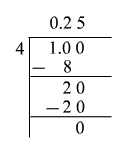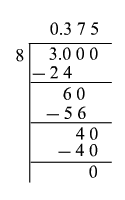# Comparing Quantities

We are familiar with comparing fractions and comparing decimals .

But what if we have to compare a fraction with a decimal? For example, is $\frac{5}{7}$ greater or less than $0.6$ ? Or, what if we have to compare a percent with a fraction? For example, is $25%$ greater or less than $\frac{2}{9}$ ?

The best way to do this is to convert both quantities to the same form. That is, convert both the quantities into a decimal or fraction or percent, and compare them.

Example 1:

Which number among $\frac{1}{4}$ and $0.3$ is greater?

Here, one number is a fraction and the other is a decimal.

To make the comparison easier, convert the fraction into a decimal. Divide the numerator by the denominator.So, $\frac{1}{4}=0.25$ .

Thus, we have two decimals, $0.25$ and $0.3$ . Since $0.3>0.25$ , the decimal $0.3$ is greater than the fraction $\frac{1}{4}$ .

Example 2:

Fill in the blanks with .

$0.4\overline{)\underset{_}{\text{\hspace{0.17em}}\text{\hspace{0.17em}}\text{\hspace{0.17em}}\text{\hspace{0.17em}}\text{\hspace{0.17em}}\text{\hspace{0.17em}}}}45%$

Here, one number is a decimal and the other is a percent.

To make the comparison easier, convert the percent into a decimal.

Remember that a percent is really just a special way of expressing a fraction as a number out of $100$ . Then, $45%=\frac{45}{100}=0.45$ .

So, $45%=0.45$ .

Thus, we have two decimals, $0.45$ and $0.4$ . Since $0.4<0.45$ , $0.4\overline{)<}45%$ .

Example 3:

Jordan finished $3$ out of $8$ homework problems. Kate is done with $40%$ of her homework problems. Who has done a greater percentage of homework?

Here, one number is a fraction and the other is a decimal.

To make the comparison easier, convert the fraction into percent. First, divide the numerator by the denominator.So, $\frac{3}{8}=0.375$ . Now, to convert to percent , multiply the decimal by $100$ .

$0.375×100=37.5$

That is, $\frac{3}{8}=37.5%$ .

Since $40%>37.5%$ , Kate has done a greater percentage of homework.